# Algebra 1 : How to subtract integers

## Example Questions

### Example Question #21 : How To Subtract Integers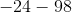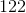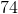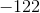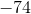Explanation:

This is the same as adding two negative numbers. When adding with another negative number, just treat as an addition problem and then add a negative sign in front. Answer is.

### Example Question #41 : Real Numbers

Subtract: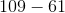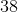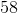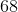Explanation:

Subtract the ones digits.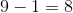Because the tens digit of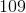is less than the tens digit of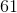, we cannot subtract the tens digit.  We need to borrow a one from the hundreds digit of.  The hundreds place becomes a zero.

Subtract: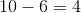The answer is:### Example Question #23 : How To Subtract Integers

Subtract: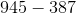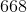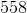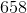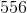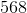Explanation:

The ones digits cannot be subtracted unless one is borrowed from the tens digt.

This becomes: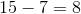This is the ones digit of the final answer.

The tens digit of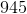becomes a.  We also cannot subtract awith thein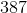, which means we will need to borrow a one form the hundreds digit of, which will become.

Subtract: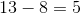This is the tens digit of the final answer.

Subtract the new hundreds digits.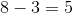This is the hundreds digit.

The answer is:### Example Question #24 : How To Subtract Integers

Find the difference between 644 and 134.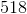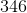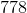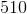Explanation:

Find the difference between 644 and 134.

Recall that difference is a clue to use subtraction. So, we need to find the following: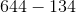We can break it down one place value at a time:

Ones: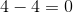Tens: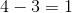Hundreds: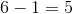### Example Question #25 : How To Subtract Integers

Subtract: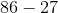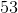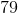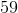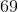Explanation:

The ones digits currently cannot be subtracted.  One must borrowed from the tens place of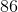.

Subtract 16 and 7.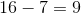This is the ones place of the final answer.

Subtract the tens digits.  Remember that since we borrowed a one, the eight becomes a seven.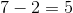Combine the tens and ones digit.

The answer is:### Example Question #26 : How To Subtract Integers

Subtract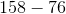.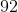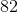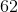Explanation:

Subtract the ones place.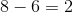Since the five in the tens place cannot subtract the seven, borrow a one from the hundreds place.  Subtract the two numbers.Combine the two numbers.

The answer is.

### Example Question #27 : How To Subtract Integers

Subtract: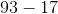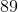Explanation:

Borrow a one from the tens digit in order to subtract the ones digits.  The tens digit become an 8.

Subtract to find the ones digit.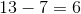Subtract the tens digits.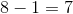The answer is:### Example Question #7 : Operations In Expressions

When evaluating the expression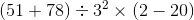,

which of the five operations must be carried out third?

Squaring

Multiplication

Subtraction

Division

Squaring

Explanation:

By the order of operations, any operations within parentheses must be carried out first; there are two here, the addition and the subtraction. After this is done, the exponent, or squaring, must be worked before the other operations. Squaring, the third operation, is therefore the correct answer.

### Example Question #8 : Operations In Expressions

When evaluating the expression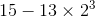,

in which order must you work the three operations?

Multiplication, cubing, subtraction

Cubing, multiplication, subtraction

Subtraction, multiplication, cubing

Cubing, subtraction, multiplication

Subtraction, cubing, multiplication

Cubing, multiplication, subtraction

Explanation:

By the order of operations, in the absence of grouping symbols, the exponent (represented here by cubing) must be worked first. The multiplication must be worked second, followed by the subtraction.

### Example Question #28 : How To Subtract Integers

Subtract the following integers: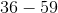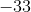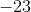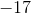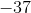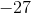Explanation:

In order to subtract these numbers, we will need to subtract 36 from 59, and then add a negative sign in front.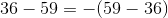Solve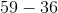.

Subtract the ones digits.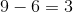Subtract the tens digits.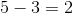The answer is: